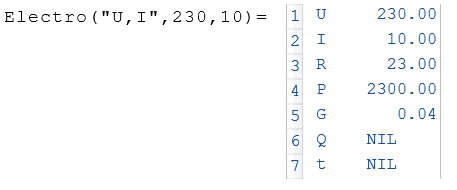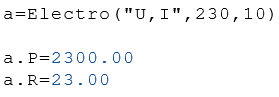# Class Electro

Calculates elementary electrical values

## Description

The class $$Electro$$ calculates elementary values of electrical engineering

## Properties

#### Description

U

Voltage in Volt

I

Current in Amperes

P

Power in Watts

R

Resistance in Ohms

G

Conductance in Siemens

Q

LCharge in Coulomb

Depending on t and I

t

Time in Seconds

Depending on Q and I

## Examples

Example 1Example 2Example 3

Electro("P->U,I",230,10)=2300